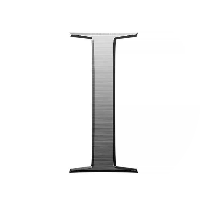# Graph Types, Properties and Other Related Concepts

This quiz contains multiple-choice problems on graph concepts like digraphs, Hasse diagrams, lattices, bipartite graphs, graph properties, connected graphs, planarity, graph colouring, different paths in graphs and graph matrices.

Start Quiz

A directed graph or digraph can have a directed cycle in which the

Starting node and ending node are different

Starting node and ending node are same

Minimum four vertices can be there

Ending node does not exist

Let D = <A, R> be a directed graph or digraph, then D’ = <A’, R’> is a subgraph if

A’ ⊂ A and R’ = R ∩ (A’ x A’)

A’ ⊂ A and R ⊂ R’ ∩ (A’ x A’)

R’ = R ∩ (A’ x A’)

A’ ⊆ A and R ⊆ R’ ∩ (A’ x A’)

The graph representing a universal relation is called

Complete digraph

Partial digraph

Empty graph

Partial subgraph

What is a complete digraph?

The connection of nodes without containing any cycle

The connection of nodes making at least three complete cycles

The start and end nodes in a graph having the same cycle

The connection of every node with every other node including itself in a digraph

Disconnected components can be created in the case of

Undirected graphs

Partial subgraphs

Disconnected graphs

Complete graphs

A simple graph can have

Multiple edges

Self loops

Parallel edges

None of the above

The degree of a graph with 12 vertices is

25

56

24

212

In a finite graph, the number of vertices of odd degree is always

Even

Odd

Even or odd

Infinite

An undirected graph has 8 vertices labelled 1, 2, …,8 and 31 edges. Vertices 1, 3, 5, 7 have degree 8 and vertices 2, 4, 6, 8 have degree 7. What is the degree of vertex 8?

15

8

5

23

G is an undirected graph with n vertices and 26 edges such that each vertex of G has a degree of at least 4. Then the maximum possible value of n is

7

43

13

10

A poset in which every pair of elements has both a least upper bound and a greatest lower bound is termed as

Sublattice

Lattice

Trail

Walk

In the poset (Z+, |) where Z+ is the set of all positive integers and | is the divides relation, are the integers 9 and 351 comparable?

Comparable

Not comparable

Comparable but not determined

Determined but not comparable

If every two elements of a poset are comparable, then the poset is called a

Subordered poset

Totally ordered poset

Sublattice

Semigroup

__ and __ are the two binary operations defined for lattices.

Join, meet

Union, intersection

Multiplication, modulo division

A __ has a greatest element and a least element which satisfy 0<=a<=1 for every a in the lattice (say, L).

Semilattice

Join semilattice

Meet semilattice

Bounded lattice

Quiz/Test Summary
Title: Graph Types, Properties and Other Related Concepts
Questions: 15
Contributed by: